Courses

# Combinations of Cells Class 12 Notes | EduRev

## JEE : Combinations of Cells Class 12 Notes | EduRev

The document Combinations of Cells Class 12 Notes | EduRev is a part of the JEE Course Physics For JEE.
All you need of JEE at this link: JEE

Battery and EMF

• A battery is a device which maintains a potential difference between its two terminals A and B.
• Figure shows a schematic diagram of a battery. Some internal mechanism exerts forces on the charges of the battery material.
• This force drives the positive charges of the battery material towards A and the negative charges of the battery material towards B. We show the force on a positive charge q as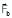. As positive charge accumulates on A and negative charge on B, a potential difference develops and grows between A and B. An electric field is developed in the battery material from A to B and exerts a force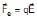on a charge q. The direction of this force is opposite to that ofIn steady state, the charge accumulation on A and B is such that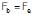. No further accumulation takes place.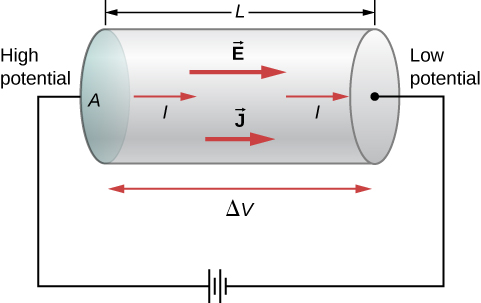• If a charge q is taken from the terminal B to the terminal A , the work done by the battery force Fb is
W = Fb d where d is the distance between A and B. The work done by the battery force per unit charge is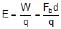• This quantity is called the emf of the battery. The full form of emf is electromotive force. The name is misleading in the sense that emf is not a force, it is work done/charge. We shall continue to denote this quantity by the short name emf. If nothing is connected externally between A and B,
Fb = Fe = qE
or, Fbd = qEd = qV
where V = Ed is the potential difference between the terminals. Thus,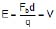• Thus, the emf of a battery equals the potential difference between its terminals when the terminals are not connected externally.
• Potential difference and emf are two different quantities whose magnitudes may be equal in certain conditions. The emf is the work done per unit charge by the battery force Fb which is non-electrostatic in nature. The potential difference originates from the electrostatic field created by the charges accumulated on the terminals of the battery.
• A battery is often prepared by putting two rods or plates of different metals in a chemical solution. Such a battery, using chemical reactions to generate emf, is often called a cell.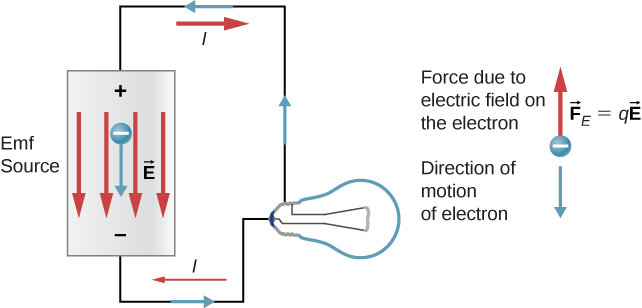• Now suppose the terminals of a battery are connected by a conducting wire as shown in above figure. As the terminal A is at a higher potential than B, there is an electric field in the wire in the direction shown in the figure.
• The free electrons in the wire move in the opposite direction .and enter the battery at the terminal A. Some electrons are withdrawn from the terminal B which enter the wire through the right end. Thus, the potential difference between A and B tends to decrease. If this potential difference decreases, the electrostatic force Fe inside the battery also decreases. The force Fb due to the battery mechanism remains the same.
• Thus, there is a net force on the positive charges of the battery material from B to A. The positive charges rush towards A and neutralize the effect of the electrons coming at A from the wire. Similarly, the negative charges rush towards B. Thus, the potential difference between A and B is maintained.
• For calculation of current, motion of a positive charge in one direction is equivalent to the motion of a negative charge in opposite direction. Using this fact, We can describe the above situation by a simpler model. The positive terminal of the battery supplies positive charges to the wire. These charges are pushed through the wire by the electric field and they reach the negative terminal of the battery. The battery mechanism drives these charges back to the positive terminal against the electric field existing in the battery and the process continues. This maintains a steady current in the circuit
• Current can also be driven into a battery in the reverse direction. In such a case, positive charge enters the battery at the positive terminal, moves inside the battery to the· negative terminal and leaves the battery from the negative terminal. Such a process is called charging of the battery. The more common process in which. the positive charge comes out of the battery from the positive terminal is called discharging of the battery.

Example 1.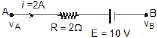Find vA - vB

Sol. vA - iR - E = vB

vA - vB = iR E = 4 +10 = 14 volt

Example 2. Shown in the figure. Find out the current in the wire BD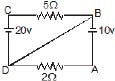Sol. Let at point D potential = 0 and write the potential of other points then current in wire AD =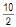= 5A from A to D current in wire CB =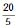= 4A from C to B. Therefore, current in wire BD = 1 A from D to B.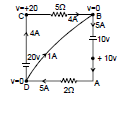Example 3.  Find the current in each wire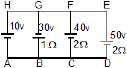Sol. Let potential at point A is 0 volt then potential of other points is shown in figure.

Current in BG =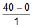= 40 A from G to B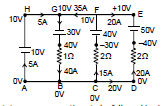Current in FC =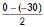= 15A from C to K

current in DE =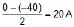from D to E

current in wire AH = 40 - 35 = 5 A from A to H

Combinations of cells

• A cell is used to maintain current in an electric circuit. We cannot obtain a strong current from a single cell.
• Hence need arises to combine two or more cells to obtain a strong current.
• Cells can be combined in three possible ways:
(i) In series
(ii) In parallel
(iii) In mixed grouping.

Cells in Series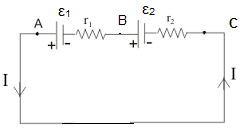• In this combination, cells are so connected that -ve terminal of each cell is connected with the +ve terminal of next and so on. Suppose n cells are connected in this way. Let e.m.f and internal resistance of each cell are E and r respectively.
• Net e.m.f of the cells = nE. Total internal resistance = nr. Hence total resistance of the circuit = nr + R.

If total current in the circuit is I, then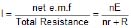...(1)

Case (i) : If nr < < R, then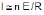i.e., if total internal resistance of the cells is far less than external resistance, then current obtained from the cells is approximately equal to n times the current obtained from a single cell. Hence cells, whose total internal resistance is less than external resistance, just be joined in series to obtain strong current.

Case (ii) : If nr >> R, then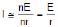i.e., if total internal resistance of the cells is much greater than the external resistance, then current obtained from the combination of n cells is nearly the same as obtained from a single cell. Hence there is no use of joining such cells in series.

Cells in Parallel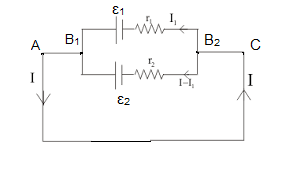➢ When E.M.F's and internal resistance of all the cells are equal

• In this combination, positive terminals of all the cells are connected at one point and negative terminals at other point. Figure shown such cells connected in parallel across some external resistance R. Let e.m.f and internal resistance of each cell are E and r respectively.
• Because all the cells are connected in parallel between two points, hence e.m.f of battery = E.
• Total internal resistance of the combination of n cells = r/n
• Because external resistance R is connected in series with internal resistance, hence total resistance of the circuit = (r/n) R
• If current in external resistance is I, then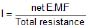=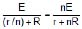Case (I) : If r << R, the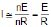i.e., if internal resistance of the cells is much less than external resistance, then total current obtained from combination is nearly equal to current given by one cells only. Hence there is no use of joining cells of low internal resistance in parallel.

Case (II) : If r >> R, then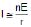i.e., if the internal resistance of the cells is much higher than the external resistance, then total current is nearly equal to n times the current given by one cell. Hence cells of high internal resistance must be joined in parallel to get a strong current.

(II) When emf's and internal resistance of all the cells connected in parallel are different.

In this case, total current in external resistance is obtained with the help of Kirchhoff's laws. Figure shows three cells of e.m.f E1, E2 and E3 and internal resistances r1, r2 and r3 connected in parallel across some external resistance R. Suppose currents given by three cells are i1, i2 and i3. Hence according to Kirchhoff's first law, total current I in external resistance R, is given by

I = i1  i2  i3 ...(1)

Applying Kirchhoff's 2nd law to closed mesh ABEF we get

IR i1r1 = E1 or i1 =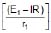...(2)

Similarly, for closed meshes ABDG and ABCH, we get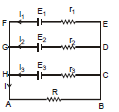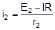....(3)

and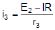....(4)

Substituting eq. (2), (3) and (4) in eq. (1), we have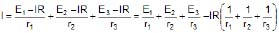or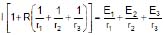or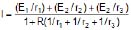If n cells are joined in parallel, then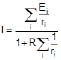and Eeq.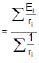, req.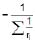Cells in Mixed Grouping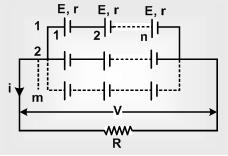In this combination, a certain number of cells are joined in series in various rows, and all such rows are then connected in parallel with each other.

Suppose n cells, each of e.m.f E and internal resistance r, are connected in series in every row and m such rows are connected in parallel across some external resistance R, as shown in figure.

Total number of cells in the combination = mn. As e.m.f. of each row = nE and all the rows are connected in parallel, hence net e.m.f of battery = nE.

Internal resistance of each row = nr. As m such rows are connected in parallel, hence total internal resistance of battery =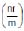Hence total resistance of the circuit =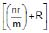If the current in external resistance is I, then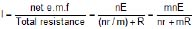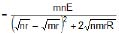It is clear from above equation that I will be maximum when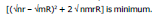This will be possible when the quantity [Önr - ÖmR]2 is minimum. Because this quantity is in square, it can not be negative, hence its minimum value will be equal to zero, i.e.,

mR = nr or R =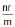Note:In mixed grouping of cells, current in external resistance will be maximum when total internal resistance of battery is equal to external resistance.

Because power consumed in the external resistance or load = I2R, hence when current in load is maximum, consumed power in it is also maximum, Hence consumed power in the load will also be maximum when R =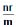.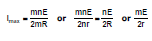Example 4. Find the current in the loop.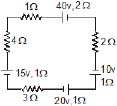Sol. The given circuit can be simplified as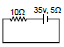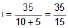=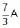⇒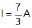Example 5. Find the emf and internal resistance of a single battery which is equivalent to a combination of three batteries as shown in figure.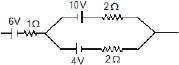Sol.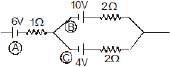Battery (B) and (C) are in parallel combination with opposite polarity. So, their equivalent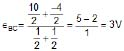rBC = 1 W

Now,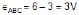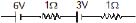rABC = 2Ω

Offer running on EduRev: Apply code STAYHOME200 to get INR 200 off on our premium plan EduRev Infinity!

## Physics For JEE

187 videos|516 docs|263 tests

,

,

,

,

,

,

,

,

,

,

,

,

,

,

,

,

,

,

,

,

,

;